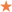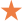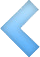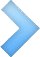020-29184661、29184662

020-29184663、29184664

PRODUCT CUSTOMIZATION

•❤ 收藏
• ## BMT80007藏青西装订做职业装定制厂家

• ￥0.00
￥0.00
￥0.00
• 满意度：成交： 0 次

评论： 0 次

重量：0.00KG

• 选择我们:不缩水
• 尺码:S
• 颜色:白色
• 选择我们:不缩水
• 尺码:S
• 颜色:红色
• 选择我们:不缩水
• 尺码:S
• 颜色:黄色
• 选择我们:不缩水
• 尺码:S
• 颜色:果绿
• 选择我们:不缩水
• 尺码:S
• 颜色:橙色
• 选择我们:不缩水
• 尺码:S
• 颜色:紫色
• 选择我们:不缩水
• 尺码:S
• 颜色:黑色
• 选择我们:不缩水
• 尺码:S
• 颜色:灰色
• 选择我们:不缩水
• 尺码:S
• 颜色:草绿
• 选择我们:不缩水
• 尺码:S
• 颜色:天蓝
• 选择我们:不缩水
• 尺码:S
• 颜色:湖蓝
• 选择我们:不缩水
• 尺码:S
• 颜色:藏蓝
• 选择我们:不缩水
• 尺码:S
• 颜色:更多颜色欢迎来定制
• 选择我们:不缩水
• 尺码:M
• 颜色:白色
• 选择我们:不缩水
• 尺码:M
• 颜色:红色
• 选择我们:不缩水
• 尺码:M
• 颜色:黄色
• 选择我们:不缩水
• 尺码:M
• 颜色:果绿
• 选择我们:不缩水
• 尺码:M
• 颜色:橙色
• 选择我们:不缩水
• 尺码:M
• 颜色:紫色
• 选择我们:不缩水
• 尺码:M
• 颜色:黑色
• 选择我们:不缩水
• 尺码:M
• 颜色:灰色
• 选择我们:不缩水
• 尺码:M
• 颜色:草绿
• 选择我们:不缩水
• 尺码:M
• 颜色:天蓝
• 选择我们:不缩水
• 尺码:M
• 颜色:湖蓝
• 选择我们:不缩水
• 尺码:M
• 颜色:藏蓝
• 选择我们:不缩水
• 尺码:M
• 颜色:更多颜色欢迎来定制
• 选择我们:不缩水
• 尺码:L
• 颜色:白色
• 选择我们:不缩水
• 尺码:L
• 颜色:红色
• 选择我们:不缩水
• 尺码:L
• 颜色:黄色
• 选择我们:不缩水
• 尺码:L
• 颜色:果绿
• 选择我们:不缩水
• 尺码:L
• 颜色:橙色
• 选择我们:不缩水
• 尺码:L
• 颜色:紫色
• 选择我们:不缩水
• 尺码:L
• 颜色:黑色
• 选择我们:不缩水
• 尺码:L
• 颜色:灰色
• 选择我们:不缩水
• 尺码:L
• 颜色:草绿
• 选择我们:不缩水
• 尺码:L
• 颜色:天蓝
• 选择我们:不缩水
• 尺码:L
• 颜色:湖蓝
• 选择我们:不缩水
• 尺码:L
• 颜色:藏蓝
• 选择我们:不缩水
• 尺码:L
• 颜色:更多颜色欢迎来定制
• 选择我们:不缩水
• 尺码:XL
• 颜色:白色
• 选择我们:不缩水
• 尺码:XL
• 颜色:红色
• 选择我们:不缩水
• 尺码:XL
• 颜色:黄色
• 选择我们:不缩水
• 尺码:XL
• 颜色:果绿
• 选择我们:不缩水
• 尺码:XL
• 颜色:橙色
• 选择我们:不缩水
• 尺码:XL
• 颜色:紫色
• 选择我们:不缩水
• 尺码:XL
• 颜色:黑色
• 选择我们:不缩水
• 尺码:XL
• 颜色:灰色
• 选择我们:不缩水
• 尺码:XL
• 颜色:草绿
• 选择我们:不缩水
• 尺码:XL
• 颜色:天蓝
• 选择我们:不缩水
• 尺码:XL
• 颜色:湖蓝
• 选择我们:不缩水
• 尺码:XL
• 颜色:藏蓝
• 选择我们:不缩水
• 尺码:XL
• 颜色:更多颜色欢迎来定制
• 选择我们:不缩水
• 尺码:XXL
• 颜色:白色
• 选择我们:不缩水
• 尺码:XXL
• 颜色:红色
• 选择我们:不缩水
• 尺码:XXL
• 颜色:黄色
• 选择我们:不缩水
• 尺码:XXL
• 颜色:果绿
• 选择我们:不缩水
• 尺码:XXL
• 颜色:橙色
• 选择我们:不缩水
• 尺码:XXL
• 颜色:紫色
• 选择我们:不缩水
• 尺码:XXL
• 颜色:黑色
• 选择我们:不缩水
• 尺码:XXL
• 颜色:灰色
• 选择我们:不缩水
• 尺码:XXL
• 颜色:草绿
• 选择我们:不缩水
• 尺码:XXL
• 颜色:天蓝
• 选择我们:不缩水
• 尺码:XXL
• 颜色:湖蓝
• 选择我们:不缩水
• 尺码:XXL
• 颜色:藏蓝
• 选择我们:不缩水
• 尺码:XXL
• 颜色:更多颜色欢迎来定制
• 选择我们:不缩水
• 尺码:XXXL
• 颜色:白色
• 选择我们:不缩水
• 尺码:XXXL
• 颜色:红色
• 选择我们:不缩水
• 尺码:XXXL
• 颜色:黄色
• 选择我们:不缩水
• 尺码:XXXL
• 颜色:果绿
• 选择我们:不缩水
• 尺码:XXXL
• 颜色:橙色
• 选择我们:不缩水
• 尺码:XXXL
• 颜色:紫色
• 选择我们:不缩水
• 尺码:XXXL
• 颜色:黑色
• 选择我们:不缩水
• 尺码:XXXL
• 颜色:灰色
• 选择我们:不缩水
• 尺码:XXXL
• 颜色:草绿
• 选择我们:不缩水
• 尺码:XXXL
• 颜色:天蓝
• 选择我们:不缩水
• 尺码:XXXL
• 颜色:湖蓝
• 选择我们:不缩水
• 尺码:XXXL
• 颜色:藏蓝
• 选择我们:不缩水
• 尺码:XXXL
• 颜色:更多颜色欢迎来定制
• 选择我们:不缩水
• 尺码:XXXXL
• 颜色:白色
• 选择我们:不缩水
• 尺码:XXXXL
• 颜色:红色
• 选择我们:不缩水
• 尺码:XXXXL
• 颜色:黄色
• 选择我们:不缩水
• 尺码:XXXXL
• 颜色:果绿
• 选择我们:不缩水
• 尺码:XXXXL
• 颜色:橙色
• 选择我们:不缩水
• 尺码:XXXXL
• 颜色:紫色
• 选择我们:不缩水
• 尺码:XXXXL
• 颜色:黑色
• 选择我们:不缩水
• 尺码:XXXXL
• 颜色:灰色
• 选择我们:不缩水
• 尺码:XXXXL
• 颜色:草绿
• 选择我们:不缩水
• 尺码:XXXXL
• 颜色:天蓝
• 选择我们:不缩水
• 尺码:XXXXL
• 颜色:湖蓝
• 选择我们:不缩水
• 尺码:XXXXL
• 颜色:藏蓝
• 选择我们:不缩水
• 尺码:XXXXL
• 颜色:更多颜色欢迎来定制
• 选择我们:不起球
• 尺码:S
• 颜色:白色
• 选择我们:不起球
• 尺码:S
• 颜色:红色
• 选择我们:不起球
• 尺码:S
• 颜色:黄色
• 选择我们:不起球
• 尺码:S
• 颜色:果绿
• 选择我们:不起球
• 尺码:S
• 颜色:橙色
• 选择我们:不起球
• 尺码:S
• 颜色:紫色
• 选择我们:不起球
• 尺码:S
• 颜色:黑色
• 选择我们:不起球
• 尺码:S
• 颜色:灰色
• 选择我们:不起球
• 尺码:S
• 颜色:草绿
• 选择我们:不起球
• 尺码:S
• 颜色:天蓝
• 选择我们:不起球
• 尺码:S
• 颜色:湖蓝
• 选择我们:不起球
• 尺码:S
• 颜色:藏蓝
• 选择我们:不起球
• 尺码:S
• 颜色:更多颜色欢迎来定制
• 选择我们:不起球
• 尺码:M
• 颜色:白色
• 选择我们:不起球
• 尺码:M
• 颜色:红色
• 选择我们:不起球
• 尺码:M
• 颜色:黄色
• 选择我们:不起球
• 尺码:M
• 颜色:果绿
• 选择我们:不起球
• 尺码:M
• 颜色:橙色
• 选择我们:不起球
• 尺码:M
• 颜色:紫色
• 选择我们:不起球
• 尺码:M
• 颜色:黑色
• 选择我们:不起球
• 尺码:M
• 颜色:灰色
• 选择我们:不起球
• 尺码:M
• 颜色:草绿
• 选择我们:不起球
• 尺码:M
• 颜色:天蓝
• 选择我们:不起球
• 尺码:M
• 颜色:湖蓝
• 选择我们:不起球
• 尺码:M
• 颜色:藏蓝
• 选择我们:不起球
• 尺码:M
• 颜色:更多颜色欢迎来定制
• 选择我们:不起球
• 尺码:L
• 颜色:白色
• 选择我们:不起球
• 尺码:L
• 颜色:红色
• 选择我们:不起球
• 尺码:L
• 颜色:黄色
• 选择我们:不起球
• 尺码:L
• 颜色:果绿
• 选择我们:不起球
• 尺码:L
• 颜色:橙色
• 选择我们:不起球
• 尺码:L
• 颜色:紫色
• 选择我们:不起球
• 尺码:L
• 颜色:黑色
• 选择我们:不起球
• 尺码:L
• 颜色:灰色
• 选择我们:不起球
• 尺码:L
• 颜色:草绿
• 选择我们:不起球
• 尺码:L
• 颜色:天蓝
• 选择我们:不起球
• 尺码:L
• 颜色:湖蓝
• 选择我们:不起球
• 尺码:L
• 颜色:藏蓝
• 选择我们:不起球
• 尺码:L
• 颜色:更多颜色欢迎来定制
• 选择我们:不起球
• 尺码:XL
• 颜色:白色
• 选择我们:不起球
• 尺码:XL
• 颜色:红色
• 选择我们:不起球
• 尺码:XL
• 颜色:黄色
• 选择我们:不起球
• 尺码:XL
• 颜色:果绿
• 选择我们:不起球
• 尺码:XL
• 颜色:橙色
• 选择我们:不起球
• 尺码:XL
• 颜色:紫色
• 选择我们:不起球
• 尺码:XL
• 颜色:黑色
• 选择我们:不起球
• 尺码:XL
• 颜色:灰色
• 选择我们:不起球
• 尺码:XL
• 颜色:草绿
• 选择我们:不起球
• 尺码:XL
• 颜色:天蓝
• 选择我们:不起球
• 尺码:XL
• 颜色:湖蓝
• 选择我们:不起球
• 尺码:XL
• 颜色:藏蓝
• 选择我们:不起球
• 尺码:XL
• 颜色:更多颜色欢迎来定制
• 选择我们:不起球
• 尺码:XXL
• 颜色:白色
• 选择我们:不起球
• 尺码:XXL
• 颜色:红色
• 选择我们:不起球
• 尺码:XXL
• 颜色:黄色
• 选择我们:不起球
• 尺码:XXL
• 颜色:果绿
• 选择我们:不起球
• 尺码:XXL
• 颜色:橙色
• 选择我们:不起球
• 尺码:XXL
• 颜色:紫色
• 选择我们:不起球
• 尺码:XXL
• 颜色:黑色
• 选择我们:不起球
• 尺码:XXL
• 颜色:灰色
• 选择我们:不起球
• 尺码:XXL
• 颜色:草绿
• 选择我们:不起球
• 尺码:XXL
• 颜色:天蓝
• 选择我们:不起球
• 尺码:XXL
• 颜色:湖蓝
• 选择我们:不起球
• 尺码:XXL
• 颜色:藏蓝
• 选择我们:不起球
• 尺码:XXL
• 颜色:更多颜色欢迎来定制
• 选择我们:不起球
• 尺码:XXXL
• 颜色:白色
• 选择我们:不起球
• 尺码:XXXL
• 颜色:红色
• 选择我们:不起球
• 尺码:XXXL
• 颜色:黄色
• 选择我们:不起球
• 尺码:XXXL
• 颜色:果绿
• 选择我们:不起球
• 尺码:XXXL
• 颜色:橙色
• 选择我们:不起球
• 尺码:XXXL
• 颜色:紫色
• 选择我们:不起球
• 尺码:XXXL
• 颜色:黑色
• 选择我们:不起球
• 尺码:XXXL
• 颜色:灰色
• 选择我们:不起球
• 尺码:XXXL
• 颜色:草绿
• 选择我们:不起球
• 尺码:XXXL
• 颜色:天蓝
• 选择我们:不起球
• 尺码:XXXL
• 颜色:湖蓝
• 选择我们:不起球
• 尺码:XXXL
• 颜色:藏蓝
• 选择我们:不起球
• 尺码:XXXL
• 颜色:更多颜色欢迎来定制
• 选择我们:不起球
• 尺码:XXXXL
• 颜色:白色
• 选择我们:不起球
• 尺码:XXXXL
• 颜色:红色
• 选择我们:不起球
• 尺码:XXXXL
• 颜色:黄色
• 选择我们:不起球
• 尺码:XXXXL
• 颜色:果绿
• 选择我们:不起球
• 尺码:XXXXL
• 颜色:橙色
• 选择我们:不起球
• 尺码:XXXXL
• 颜色:紫色
• 选择我们:不起球
• 尺码:XXXXL
• 颜色:黑色
• 选择我们:不起球
• 尺码:XXXXL
• 颜色:灰色
• 选择我们:不起球
• 尺码:XXXXL
• 颜色:草绿
• 选择我们:不起球
• 尺码:XXXXL
• 颜色:天蓝
• 选择我们:不起球
• 尺码:XXXXL
• 颜色:湖蓝
• 选择我们:不起球
• 尺码:XXXXL
• 颜色:藏蓝
• 选择我们:不起球
• 尺码:XXXXL
• 颜色:更多颜色欢迎来定制
• 选择我们:不掉色
• 尺码:S
• 颜色:白色
• 选择我们:不掉色
• 尺码:S
• 颜色:红色
• 选择我们:不掉色
• 尺码:S
• 颜色:黄色
• 选择我们:不掉色
• 尺码:S
• 颜色:果绿
• 选择我们:不掉色
• 尺码:S
• 颜色:橙色
• 选择我们:不掉色
• 尺码:S
• 颜色:紫色
• 选择我们:不掉色
• 尺码:S
• 颜色:黑色
• 选择我们:不掉色
• 尺码:S
• 颜色:灰色
• 选择我们:不掉色
• 尺码:S
• 颜色:草绿
• 选择我们:不掉色
• 尺码:S
• 颜色:天蓝
• 选择我们:不掉色
• 尺码:S
• 颜色:湖蓝
• 选择我们:不掉色
• 尺码:S
• 颜色:藏蓝
• 选择我们:不掉色
• 尺码:S
• 颜色:更多颜色欢迎来定制
• 选择我们:不掉色
• 尺码:M
• 颜色:白色
• 选择我们:不掉色
• 尺码:M
• 颜色:红色
• 选择我们:不掉色
• 尺码:M
• 颜色:黄色
• 选择我们:不掉色
• 尺码:M
• 颜色:果绿
• 选择我们:不掉色
• 尺码:M
• 颜色:橙色
• 选择我们:不掉色
• 尺码:M
• 颜色:紫色
• 选择我们:不掉色
• 尺码:M
• 颜色:黑色
• 选择我们:不掉色
• 尺码:M
• 颜色:灰色
• 选择我们:不掉色
• 尺码:M
• 颜色:草绿
• 选择我们:不掉色
• 尺码:M
• 颜色:天蓝
• 选择我们:不掉色
• 尺码:M
• 颜色:湖蓝
• 选择我们:不掉色
• 尺码:M
• 颜色:藏蓝
• 选择我们:不掉色
• 尺码:M
• 颜色:更多颜色欢迎来定制
• 选择我们:不掉色
• 尺码:L
• 颜色:白色
• 选择我们:不掉色
• 尺码:L
• 颜色:红色
• 选择我们:不掉色
• 尺码:L
• 颜色:黄色
• 选择我们:不掉色
• 尺码:L
• 颜色:果绿
• 选择我们:不掉色
• 尺码:L
• 颜色:橙色
• 选择我们:不掉色
• 尺码:L
• 颜色:紫色
• 选择我们:不掉色
• 尺码:L
• 颜色:黑色
• 选择我们:不掉色
• 尺码:L
• 颜色:灰色
• 选择我们:不掉色
• 尺码:L
• 颜色:草绿
• 选择我们:不掉色
• 尺码:L
• 颜色:天蓝
• 选择我们:不掉色
• 尺码:L
• 颜色:湖蓝
• 选择我们:不掉色
• 尺码:L
• 颜色:藏蓝
• 选择我们:不掉色
• 尺码:L
• 颜色:更多颜色欢迎来定制
• 选择我们:不掉色
• 尺码:XL
• 颜色:白色
• 选择我们:不掉色
• 尺码:XL
• 颜色:红色
• 选择我们:不掉色
• 尺码:XL
• 颜色:黄色
• 选择我们:不掉色
• 尺码:XL
• 颜色:果绿
• 选择我们:不掉色
• 尺码:XL
• 颜色:橙色
• 选择我们:不掉色
• 尺码:XL
• 颜色:紫色
• 选择我们:不掉色
• 尺码:XL
• 颜色:黑色
• 选择我们:不掉色
• 尺码:XL
• 颜色:灰色
• 选择我们:不掉色
• 尺码:XL
• 颜色:草绿
• 选择我们:不掉色
• 尺码:XL
• 颜色:天蓝
• 选择我们:不掉色
• 尺码:XL
• 颜色:湖蓝
• 选择我们:不掉色
• 尺码:XL
• 颜色:藏蓝
• 选择我们:不掉色
• 尺码:XL
• 颜色:更多颜色欢迎来定制
• 选择我们:不掉色
• 尺码:XXL
• 颜色:白色
• 选择我们:不掉色
• 尺码:XXL
• 颜色:红色
• 选择我们:不掉色
• 尺码:XXL
• 颜色:黄色
• 选择我们:不掉色
• 尺码:XXL
• 颜色:果绿
• 选择我们:不掉色
• 尺码:XXL
• 颜色:橙色
• 选择我们:不掉色
• 尺码:XXL
• 颜色:紫色
• 选择我们:不掉色
• 尺码:XXL
• 颜色:黑色
• 选择我们:不掉色
• 尺码:XXL
• 颜色:灰色
• 选择我们:不掉色
• 尺码:XXL
• 颜色:草绿
• 选择我们:不掉色
• 尺码:XXL
• 颜色:天蓝
• 选择我们:不掉色
• 尺码:XXL
• 颜色:湖蓝
• 选择我们:不掉色
• 尺码:XXL
• 颜色:藏蓝
• 选择我们:不掉色
• 尺码:XXL
• 颜色:更多颜色欢迎来定制
• 选择我们:不掉色
• 尺码:XXXL
• 颜色:白色
• 选择我们:不掉色
• 尺码:XXXL
• 颜色:红色
• 选择我们:不掉色
• 尺码:XXXL
• 颜色:黄色
• 选择我们:不掉色
• 尺码:XXXL
• 颜色:果绿
• 选择我们:不掉色
• 尺码:XXXL
• 颜色:橙色
• 选择我们:不掉色
• 尺码:XXXL
• 颜色:紫色
• 选择我们:不掉色
• 尺码:XXXL
• 颜色:黑色
• 选择我们:不掉色
• 尺码:XXXL
• 颜色:灰色
• 选择我们:不掉色
• 尺码:XXXL
• 颜色:草绿
• 选择我们:不掉色
• 尺码:XXXL
• 颜色:天蓝
• 选择我们:不掉色
• 尺码:XXXL
• 颜色:湖蓝
• 选择我们:不掉色
• 尺码:XXXL
• 颜色:藏蓝
• 选择我们:不掉色
• 尺码:XXXL
• 颜色:更多颜色欢迎来定制
• 选择我们:不掉色
• 尺码:XXXXL
• 颜色:白色
• 选择我们:不掉色
• 尺码:XXXXL
• 颜色:红色
• 选择我们:不掉色
• 尺码:XXXXL
• 颜色:黄色
• 选择我们:不掉色
• 尺码:XXXXL
• 颜色:果绿
• 选择我们:不掉色
• 尺码:XXXXL
• 颜色:橙色
• 选择我们:不掉色
• 尺码:XXXXL
• 颜色:紫色
• 选择我们:不掉色
• 尺码:XXXXL
• 颜色:黑色
• 选择我们:不掉色
• 尺码:XXXXL
• 颜色:灰色
• 选择我们:不掉色
• 尺码:XXXXL
• 颜色:草绿
• 选择我们:不掉色
• 尺码:XXXXL
• 颜色:天蓝
• 选择我们:不掉色
• 尺码:XXXXL
• 颜色:湖蓝
• 选择我们:不掉色
• 尺码:XXXXL
• 颜色:藏蓝
• 选择我们:不掉色
• 尺码:XXXXL
• 颜色:更多颜色欢迎来定制
• 选择我们:环保定染
• 尺码:S
• 颜色:白色
• 选择我们:环保定染
• 尺码:S
• 颜色:红色
• 选择我们:环保定染
• 尺码:S
• 颜色:黄色
• 选择我们:环保定染
• 尺码:S
• 颜色:果绿
• 选择我们:环保定染
• 尺码:S
• 颜色:橙色
• 选择我们:环保定染
• 尺码:S
• 颜色:紫色
• 选择我们:环保定染
• 尺码:S
• 颜色:黑色
• 选择我们:环保定染
• 尺码:S
• 颜色:灰色
• 选择我们:环保定染
• 尺码:S
• 颜色:草绿
• 选择我们:环保定染
• 尺码:S
• 颜色:天蓝
• 选择我们:环保定染
• 尺码:S
• 颜色:湖蓝
• 选择我们:环保定染
• 尺码:S
• 颜色:藏蓝
• 选择我们:环保定染
• 尺码:S
• 颜色:更多颜色欢迎来定制
• 选择我们:环保定染
• 尺码:M
• 颜色:白色
• 选择我们:环保定染
• 尺码:M
• 颜色:红色
• 选择我们:环保定染
• 尺码:M
• 颜色:黄色
• 选择我们:环保定染
• 尺码:M
• 颜色:果绿
• 选择我们:环保定染
• 尺码:M
• 颜色:橙色
• 选择我们:环保定染
• 尺码:M
• 颜色:紫色
• 选择我们:环保定染
• 尺码:M
• 颜色:黑色
• 选择我们:环保定染
• 尺码:M
• 颜色:灰色
• 选择我们:环保定染
• 尺码:M
• 颜色:草绿
• 选择我们:环保定染
• 尺码:M
• 颜色:天蓝
• 选择我们:环保定染
• 尺码:M
• 颜色:湖蓝
• 选择我们:环保定染
• 尺码:M
• 颜色:藏蓝
• 选择我们:环保定染
• 尺码:M
• 颜色:更多颜色欢迎来定制
• 选择我们:环保定染
• 尺码:L
• 颜色:白色
• 选择我们:环保定染
• 尺码:L
• 颜色:红色
• 选择我们:环保定染
• 尺码:L
• 颜色:黄色
• 选择我们:环保定染
• 尺码:L
• 颜色:果绿
• 选择我们:环保定染
• 尺码:L
• 颜色:橙色
• 选择我们:环保定染
• 尺码:L
• 颜色:紫色
• 选择我们:环保定染
• 尺码:L
• 颜色:黑色
• 选择我们:环保定染
• 尺码:L
• 颜色:灰色
• 选择我们:环保定染
• 尺码:L
• 颜色:草绿
• 选择我们:环保定染
• 尺码:L
• 颜色:天蓝
• 选择我们:环保定染
• 尺码:L
• 颜色:湖蓝
• 选择我们:环保定染
• 尺码:L
• 颜色:藏蓝
• 选择我们:环保定染
• 尺码:L
• 颜色:更多颜色欢迎来定制
• 选择我们:环保定染
• 尺码:XL
• 颜色:白色
• 选择我们:环保定染
• 尺码:XL
• 颜色:红色
• 选择我们:环保定染
• 尺码:XL
• 颜色:黄色
• 选择我们:环保定染
• 尺码:XL
• 颜色:果绿
• 选择我们:环保定染
• 尺码:XL
• 颜色:橙色
• 选择我们:环保定染
• 尺码:XL
• 颜色:紫色
• 选择我们:环保定染
• 尺码:XL
• 颜色:黑色
• 选择我们:环保定染
• 尺码:XL
• 颜色:灰色
• 选择我们:环保定染
• 尺码:XL
• 颜色:草绿
• 选择我们:环保定染
• 尺码:XL
• 颜色:天蓝
• 选择我们:环保定染
• 尺码:XL
• 颜色:湖蓝
• 选择我们:环保定染
• 尺码:XL
• 颜色:藏蓝
• 选择我们:环保定染
• 尺码:XL
• 颜色:更多颜色欢迎来定制
• 选择我们:环保定染
• 尺码:XXL
• 颜色:白色
• 选择我们:环保定染
• 尺码:XXL
• 颜色:红色
• 选择我们:环保定染
• 尺码:XXL
• 颜色:黄色
• 选择我们:环保定染
• 尺码:XXL
• 颜色:果绿
• 选择我们:环保定染
• 尺码:XXL
• 颜色:橙色
• 选择我们:环保定染
• 尺码:XXL
• 颜色:紫色
• 选择我们:环保定染
• 尺码:XXL
• 颜色:黑色
• 选择我们:环保定染
• 尺码:XXL
• 颜色:灰色
• 选择我们:环保定染
• 尺码:XXL
• 颜色:草绿
• 选择我们:环保定染
• 尺码:XXL
• 颜色:天蓝
• 选择我们:环保定染
• 尺码:XXL
• 颜色:湖蓝
• 选择我们:环保定染
• 尺码:XXL
• 颜色:藏蓝
• 选择我们:环保定染
• 尺码:XXL
• 颜色:更多颜色欢迎来定制
• 选择我们:环保定染
• 尺码:XXXL
• 颜色:白色
• 选择我们:环保定染
• 尺码:XXXL
• 颜色:红色
• 选择我们:环保定染
• 尺码:XXXL
• 颜色:黄色
• 选择我们:环保定染
• 尺码:XXXL
• 颜色:果绿
• 选择我们:环保定染
• 尺码:XXXL
• 颜色:橙色
• 选择我们:环保定染
• 尺码:XXXL
• 颜色:紫色
• 选择我们:环保定染
• 尺码:XXXL
• 颜色:黑色
• 选择我们:环保定染
• 尺码:XXXL
• 颜色:灰色
• 选择我们:环保定染
• 尺码:XXXL
• 颜色:草绿
• 选择我们:环保定染
• 尺码:XXXL
• 颜色:天蓝
• 选择我们:环保定染
• 尺码:XXXL
• 颜色:湖蓝
• 选择我们:环保定染
• 尺码:XXXL
• 颜色:藏蓝
• 选择我们:环保定染
• 尺码:XXXL
• 颜色:更多颜色欢迎来定制
• 选择我们:环保定染
• 尺码:XXXXL
• 颜色:白色
• 选择我们:环保定染
• 尺码:XXXXL
• 颜色:红色
• 选择我们:环保定染
• 尺码:XXXXL
• 颜色:黄色
• 选择我们:环保定染
• 尺码:XXXXL
• 颜色:果绿
• 选择我们:环保定染
• 尺码:XXXXL
• 颜色:橙色
• 选择我们:环保定染
• 尺码:XXXXL
• 颜色:紫色
• 选择我们:环保定染
• 尺码:XXXXL
• 颜色:黑色
• 选择我们:环保定染
• 尺码:XXXXL
• 颜色:灰色
• 选择我们:环保定染
• 尺码:XXXXL
• 颜色:草绿
• 选择我们:环保定染
• 尺码:XXXXL
• 颜色:天蓝
• 选择我们:环保定染
• 尺码:XXXXL
• 颜色:湖蓝
• 选择我们:环保定染
• 尺码:XXXXL
• 颜色:藏蓝
• 选择我们:环保定染
• 尺码:XXXXL
• 颜色:更多颜色欢迎来定制
• 选择我们:缩水定型
• 尺码:S
• 颜色:白色
• 选择我们:缩水定型
• 尺码:S
• 颜色:红色
• 选择我们:缩水定型
• 尺码:S
• 颜色:黄色
• 选择我们:缩水定型
• 尺码:S
• 颜色:果绿
• 选择我们:缩水定型
• 尺码:S
• 颜色:橙色
• 选择我们:缩水定型
• 尺码:S
• 颜色:紫色
• 选择我们:缩水定型
• 尺码:S
• 颜色:黑色
• 选择我们:缩水定型
• 尺码:S
• 颜色:灰色
• 选择我们:缩水定型
• 尺码:S
• 颜色:草绿
• 选择我们:缩水定型
• 尺码:S
• 颜色:天蓝
• 选择我们:缩水定型
• 尺码:S
• 颜色:湖蓝
• 选择我们:缩水定型
• 尺码:S
• 颜色:藏蓝
• 选择我们:缩水定型
• 尺码:S
• 颜色:更多颜色欢迎来定制
• 选择我们:缩水定型
• 尺码:M
• 颜色:白色
• 选择我们:缩水定型
• 尺码:M
• 颜色:红色
• 选择我们:缩水定型
• 尺码:M
• 颜色:黄色
• 选择我们:缩水定型
• 尺码:M
• 颜色:果绿
• 选择我们:缩水定型
• 尺码:M
• 颜色:橙色
• 选择我们:缩水定型
• 尺码:M
• 颜色:紫色
• 选择我们:缩水定型
• 尺码:M
• 颜色:黑色
• 选择我们:缩水定型
• 尺码:M
• 颜色:灰色
• 选择我们:缩水定型
• 尺码:M
• 颜色:草绿
• 选择我们:缩水定型
• 尺码:M
• 颜色:天蓝
• 选择我们:缩水定型
• 尺码:M
• 颜色:湖蓝
• 选择我们:缩水定型
• 尺码:M
• 颜色:藏蓝
• 选择我们:缩水定型
• 尺码:M
• 颜色:更多颜色欢迎来定制
• 选择我们:缩水定型
• 尺码:L
• 颜色:白色
• 选择我们:缩水定型
• 尺码:L
• 颜色:红色
• 选择我们:缩水定型
• 尺码:L
• 颜色:黄色
• 选择我们:缩水定型
• 尺码:L
• 颜色:果绿
• 选择我们:缩水定型
• 尺码:L
• 颜色:橙色
• 选择我们:缩水定型
• 尺码:L
• 颜色:紫色
• 选择我们:缩水定型
• 尺码:L
• 颜色:黑色
• 选择我们:缩水定型
• 尺码:L
• 颜色:灰色
• 选择我们:缩水定型
• 尺码:L
• 颜色:草绿
• 选择我们:缩水定型
• 尺码:L
• 颜色:天蓝
• 选择我们:缩水定型
• 尺码:L
• 颜色:湖蓝
• 选择我们:缩水定型
• 尺码:L
• 颜色:藏蓝
• 选择我们:缩水定型
• 尺码:L
• 颜色:更多颜色欢迎来定制
• 选择我们:缩水定型
• 尺码:XL
• 颜色:白色
• 选择我们:缩水定型
• 尺码:XL
• 颜色:红色
• 选择我们:缩水定型
• 尺码:XL
• 颜色:黄色
• 选择我们:缩水定型
• 尺码:XL
• 颜色:果绿
• 选择我们:缩水定型
• 尺码:XL
• 颜色:橙色
• 选择我们:缩水定型
• 尺码:XL
• 颜色:紫色
• 选择我们:缩水定型
• 尺码:XL
• 颜色:黑色
• 选择我们:缩水定型
• 尺码:XL
• 颜色:灰色
• 选择我们:缩水定型
• 尺码:XL
• 颜色:草绿
• 选择我们:缩水定型
• 尺码:XL
• 颜色:天蓝
• 选择我们:缩水定型
• 尺码:XL
• 颜色:湖蓝
• 选择我们:缩水定型
• 尺码:XL
• 颜色:藏蓝
• 选择我们:缩水定型
• 尺码:XL
• 颜色:更多颜色欢迎来定制
• 选择我们:缩水定型
• 尺码:XXL
• 颜色:白色
• 选择我们:缩水定型
• 尺码:XXL
• 颜色:红色
• 选择我们:缩水定型
• 尺码:XXL
• 颜色:黄色
• 选择我们:缩水定型
• 尺码:XXL
• 颜色:果绿
• 选择我们:缩水定型
• 尺码:XXL
• 颜色:橙色
• 选择我们:缩水定型
• 尺码:XXL
• 颜色:紫色
• 选择我们:缩水定型
• 尺码:XXL
• 颜色:黑色
• 选择我们:缩水定型
• 尺码:XXL
• 颜色:灰色
• 选择我们:缩水定型
• 尺码:XXL
• 颜色:草绿
• 选择我们:缩水定型
• 尺码:XXL
• 颜色:天蓝
• 选择我们:缩水定型
• 尺码:XXL
• 颜色:湖蓝
• 选择我们:缩水定型
• 尺码:XXL
• 颜色:藏蓝
• 选择我们:缩水定型
• 尺码:XXL
• 颜色:更多颜色欢迎来定制
• 选择我们:缩水定型
• 尺码:XXXL
• 颜色:白色
• 选择我们:缩水定型
• 尺码:XXXL
• 颜色:红色
• 选择我们:缩水定型
• 尺码:XXXL
• 颜色:黄色
• 选择我们:缩水定型
• 尺码:XXXL
• 颜色:果绿
• 选择我们:缩水定型
• 尺码:XXXL
• 颜色:橙色
• 选择我们:缩水定型
• 尺码:XXXL
• 颜色:紫色
• 选择我们:缩水定型
• 尺码:XXXL
• 颜色:黑色
• 选择我们:缩水定型
• 尺码:XXXL
• 颜色:灰色
• 选择我们:缩水定型
• 尺码:XXXL
• 颜色:草绿
• 选择我们:缩水定型
• 尺码:XXXL
• 颜色:天蓝
• 选择我们:缩水定型
• 尺码:XXXL
• 颜色:湖蓝
• 选择我们:缩水定型
• 尺码:XXXL
• 颜色:藏蓝
• 选择我们:缩水定型
• 尺码:XXXL
• 颜色:更多颜色欢迎来定制
• 选择我们:缩水定型
• 尺码:XXXXL
• 颜色:白色
• 选择我们:缩水定型
• 尺码:XXXXL
• 颜色:红色
• 选择我们:缩水定型
• 尺码:XXXXL
• 颜色:黄色
• 选择我们:缩水定型
• 尺码:XXXXL
• 颜色:果绿
• 选择我们:缩水定型
• 尺码:XXXXL
• 颜色:橙色
• 选择我们:缩水定型
• 尺码:XXXXL
• 颜色:紫色
• 选择我们:缩水定型
• 尺码:XXXXL
• 颜色:黑色
• 选择我们:缩水定型
• 尺码:XXXXL
• 颜色:灰色
• 选择我们:缩水定型
• 尺码:XXXXL
• 颜色:草绿
• 选择我们:缩水定型
• 尺码:XXXXL
• 颜色:天蓝
• 选择我们:缩水定型
• 尺码:XXXXL
• 颜色:湖蓝
• 选择我们:缩水定型
• 尺码:XXXXL
• 颜色:藏蓝
• 选择我们:缩水定型
• 尺码:XXXXL
• 颜色:更多颜色欢迎来定制
• 选择我们: 流水车间缝制
• 尺码:S
• 颜色:白色
• 选择我们: 流水车间缝制
• 尺码:S
• 颜色:红色
• 选择我们: 流水车间缝制
• 尺码:S
• 颜色:黄色
• 选择我们: 流水车间缝制
• 尺码:S
• 颜色:果绿
• 选择我们: 流水车间缝制
• 尺码:S
• 颜色:橙色
• 选择我们: 流水车间缝制
• 尺码:S
• 颜色:紫色
• 选择我们: 流水车间缝制
• 尺码:S
• 颜色:黑色
• 选择我们: 流水车间缝制
• 尺码:S
• 颜色:灰色
• 选择我们: 流水车间缝制
• 尺码:S
• 颜色:草绿
• 选择我们: 流水车间缝制
• 尺码:S
• 颜色:天蓝
• 选择我们: 流水车间缝制
• 尺码:S
• 颜色:湖蓝
• 选择我们: 流水车间缝制
• 尺码:S
• 颜色:藏蓝
• 选择我们: 流水车间缝制
• 尺码:S
• 颜色:更多颜色欢迎来定制
• 选择我们: 流水车间缝制
• 尺码:M
• 颜色:白色
• 选择我们: 流水车间缝制
• 尺码:M
• 颜色:红色
• 选择我们: 流水车间缝制
• 尺码:M
• 颜色:黄色
• 选择我们: 流水车间缝制
• 尺码:M
• 颜色:果绿
• 选择我们: 流水车间缝制
• 尺码:M
• 颜色:橙色
• 选择我们: 流水车间缝制
• 尺码:M
• 颜色:紫色
• 选择我们: 流水车间缝制
• 尺码:M
• 颜色:黑色
• 选择我们: 流水车间缝制
• 尺码:M
• 颜色:灰色
• 选择我们: 流水车间缝制
• 尺码:M
• 颜色:草绿
• 选择我们: 流水车间缝制
• 尺码:M
• 颜色:天蓝
• 选择我们: 流水车间缝制
• 尺码:M
• 颜色:湖蓝
• 选择我们: 流水车间缝制
• 尺码:M
• 颜色:藏蓝
• 选择我们: 流水车间缝制
• 尺码:M
• 颜色:更多颜色欢迎来定制
• 选择我们: 流水车间缝制
• 尺码:L
• 颜色:白色
• 选择我们: 流水车间缝制
• 尺码:L
• 颜色:红色
• 选择我们: 流水车间缝制
• 尺码:L
• 颜色:黄色
• 选择我们: 流水车间缝制
• 尺码:L
• 颜色:果绿
• 选择我们: 流水车间缝制
• 尺码:L
• 颜色:橙色
• 选择我们: 流水车间缝制
• 尺码:L
• 颜色:紫色
• 选择我们: 流水车间缝制
• 尺码:L
• 颜色:黑色
• 选择我们: 流水车间缝制
• 尺码:L
• 颜色:灰色
• 选择我们: 流水车间缝制
• 尺码:L
• 颜色:草绿
• 选择我们: 流水车间缝制
• 尺码:L
• 颜色:天蓝
• 选择我们: 流水车间缝制
• 尺码:L
• 颜色:湖蓝
• 选择我们: 流水车间缝制
• 尺码:L
• 颜色:藏蓝
• 选择我们: 流水车间缝制
• 尺码:L
• 颜色:更多颜色欢迎来定制
• 选择我们: 流水车间缝制
• 尺码:XL
• 颜色:白色
• 选择我们: 流水车间缝制
• 尺码:XL
• 颜色:红色
• 选择我们: 流水车间缝制
• 尺码:XL
• 颜色:黄色
• 选择我们: 流水车间缝制
• 尺码:XL
• 颜色:果绿
• 选择我们: 流水车间缝制
• 尺码:XL
• 颜色:橙色
• 选择我们: 流水车间缝制
• 尺码:XL
• 颜色:紫色
• 选择我们: 流水车间缝制
• 尺码:XL
• 颜色:黑色
• 选择我们: 流水车间缝制
• 尺码:XL
• 颜色:灰色
• 选择我们: 流水车间缝制
• 尺码:XL
• 颜色:草绿
• 选择我们: 流水车间缝制
• 尺码:XL
• 颜色:天蓝
• 选择我们: 流水车间缝制
• 尺码:XL
• 颜色:湖蓝
• 选择我们: 流水车间缝制
• 尺码:XL
• 颜色:藏蓝
• 选择我们: 流水车间缝制
• 尺码:XL
• 颜色:更多颜色欢迎来定制
• 选择我们: 流水车间缝制
• 尺码:XXL
• 颜色:白色
• 选择我们: 流水车间缝制
• 尺码:XXL
• 颜色:红色
• 选择我们: 流水车间缝制
• 尺码:XXL
• 颜色:黄色
• 选择我们: 流水车间缝制
• 尺码:XXL
• 颜色:果绿
• 选择我们: 流水车间缝制
• 尺码:XXL
• 颜色:橙色
• 选择我们: 流水车间缝制
• 尺码:XXL
• 颜色:紫色
• 选择我们: 流水车间缝制
• 尺码:XXL
• 颜色:黑色
• 选择我们: 流水车间缝制
• 尺码:XXL
• 颜色:灰色
• 选择我们: 流水车间缝制
• 尺码:XXL
• 颜色:草绿
• 选择我们: 流水车间缝制
• 尺码:XXL
• 颜色:天蓝
• 选择我们: 流水车间缝制
• 尺码:XXL
• 颜色:湖蓝
• 选择我们: 流水车间缝制
• 尺码:XXL
• 颜色:藏蓝
• 选择我们: 流水车间缝制
• 尺码:XXL
• 颜色:更多颜色欢迎来定制
• 选择我们: 流水车间缝制
• 尺码:XXXL
• 颜色:白色
• 选择我们: 流水车间缝制
• 尺码:XXXL
• 颜色:红色
• 选择我们: 流水车间缝制
• 尺码:XXXL
• 颜色:黄色
• 选择我们: 流水车间缝制
• 尺码:XXXL
• 颜色:果绿
• 选择我们: 流水车间缝制
• 尺码:XXXL
• 颜色:橙色
• 选择我们: 流水车间缝制
• 尺码:XXXL
• 颜色:紫色
• 选择我们: 流水车间缝制
• 尺码:XXXL
• 颜色:黑色
• 选择我们: 流水车间缝制
• 尺码:XXXL
• 颜色:灰色
• 选择我们: 流水车间缝制
• 尺码:XXXL
• 颜色:草绿
• 选择我们: 流水车间缝制
• 尺码:XXXL
• 颜色:天蓝
• 选择我们: 流水车间缝制
• 尺码:XXXL
• 颜色:湖蓝
• 选择我们: 流水车间缝制
• 尺码:XXXL
• 颜色:藏蓝
• 选择我们: 流水车间缝制
• 尺码:XXXL
• 颜色:更多颜色欢迎来定制
• 选择我们: 流水车间缝制
• 尺码:XXXXL
• 颜色:白色
• 选择我们: 流水车间缝制
• 尺码:XXXXL
• 颜色:红色
• 选择我们: 流水车间缝制
• 尺码:XXXXL
• 颜色:黄色
• 选择我们: 流水车间缝制
• 尺码:XXXXL
• 颜色:果绿
• 选择我们: 流水车间缝制
• 尺码:XXXXL
• 颜色:橙色
• 选择我们: 流水车间缝制
• 尺码:XXXXL
• 颜色:紫色
• 选择我们: 流水车间缝制
• 尺码:XXXXL
• 颜色:黑色
• 选择我们: 流水车间缝制
• 尺码:XXXXL
• 颜色:灰色
• 选择我们: 流水车间缝制
• 尺码:XXXXL
• 颜色:草绿
• 选择我们: 流水车间缝制
• 尺码:XXXXL
• 颜色:天蓝
• 选择我们: 流水车间缝制
• 尺码:XXXXL
• 颜色:湖蓝
• 选择我们: 流水车间缝制
• 尺码:XXXXL
• 颜色:藏蓝
• 选择我们: 流水车间缝制
• 尺码:XXXXL
• 颜色:更多颜色欢迎来定制
• 选择我们:人体工学裁剪
• 尺码:S
• 颜色:白色
• 选择我们:人体工学裁剪
• 尺码:S
• 颜色:红色
• 选择我们:人体工学裁剪
• 尺码:S
• 颜色:黄色
• 选择我们:人体工学裁剪
• 尺码:S
• 颜色:果绿
• 选择我们:人体工学裁剪
• 尺码:S
• 颜色:橙色
• 选择我们:人体工学裁剪
• 尺码:S
• 颜色:紫色
• 选择我们:人体工学裁剪
• 尺码:S
• 颜色:黑色
• 选择我们:人体工学裁剪
• 尺码:S
• 颜色:灰色
• 选择我们:人体工学裁剪
• 尺码:S
• 颜色:草绿
• 选择我们:人体工学裁剪
• 尺码:S
• 颜色:天蓝
• 选择我们:人体工学裁剪
• 尺码:S
• 颜色:湖蓝
• 选择我们:人体工学裁剪
• 尺码:S
• 颜色:藏蓝
• 选择我们:人体工学裁剪
• 尺码:S
• 颜色:更多颜色欢迎来定制
• 选择我们:人体工学裁剪
• 尺码:M
• 颜色:白色
• 选择我们:人体工学裁剪
• 尺码:M
• 颜色:红色
• 选择我们:人体工学裁剪
• 尺码:M
• 颜色:黄色
• 选择我们:人体工学裁剪
• 尺码:M
• 颜色:果绿
• 选择我们:人体工学裁剪
• 尺码:M
• 颜色:橙色
• 选择我们:人体工学裁剪
• 尺码:M
• 颜色:紫色
• 选择我们:人体工学裁剪
• 尺码:M
• 颜色:黑色
• 选择我们:人体工学裁剪
• 尺码:M
• 颜色:灰色
• 选择我们:人体工学裁剪
• 尺码:M
• 颜色:草绿
• 选择我们:人体工学裁剪
• 尺码:M
• 颜色:天蓝
• 选择我们:人体工学裁剪
• 尺码:M
• 颜色:湖蓝
• 选择我们:人体工学裁剪
• 尺码:M
• 颜色:藏蓝
• 选择我们:人体工学裁剪
• 尺码:M
• 颜色:更多颜色欢迎来定制
• 选择我们:人体工学裁剪
• 尺码:L
• 颜色:白色
• 选择我们:人体工学裁剪
• 尺码:L
• 颜色:红色
• 选择我们:人体工学裁剪
• 尺码:L
• 颜色:黄色
• 选择我们:人体工学裁剪
• 尺码:L
• 颜色:果绿
• 选择我们:人体工学裁剪
• 尺码:L
• 颜色:橙色
• 选择我们:人体工学裁剪
• 尺码:L
• 颜色:紫色
• 选择我们:人体工学裁剪
• 尺码:L
• 颜色:黑色
• 选择我们:人体工学裁剪
• 尺码:L
• 颜色:灰色
• 选择我们:人体工学裁剪
• 尺码:L
• 颜色:草绿
• 选择我们:人体工学裁剪
• 尺码:L
• 颜色:天蓝
• 选择我们:人体工学裁剪
• 尺码:L
• 颜色:湖蓝
• 选择我们:人体工学裁剪
• 尺码:L
• 颜色:藏蓝
• 选择我们:人体工学裁剪
• 尺码:L
• 颜色:更多颜色欢迎来定制
• 选择我们:人体工学裁剪
• 尺码:XL
• 颜色:白色
• 选择我们:人体工学裁剪
• 尺码:XL
• 颜色:红色
• 选择我们:人体工学裁剪
• 尺码:XL
• 颜色:黄色
• 选择我们:人体工学裁剪
• 尺码:XL
• 颜色:果绿
• 选择我们:人体工学裁剪
• 尺码:XL
• 颜色:橙色
• 选择我们:人体工学裁剪
• 尺码:XL
• 颜色:紫色
• 选择我们:人体工学裁剪
• 尺码:XL
• 颜色:黑色
• 选择我们:人体工学裁剪
• 尺码:XL
• 颜色:灰色
• 选择我们:人体工学裁剪
• 尺码:XL
• 颜色:草绿
• 选择我们:人体工学裁剪
• 尺码:XL
• 颜色:天蓝
• 选择我们:人体工学裁剪
• 尺码:XL
• 颜色:湖蓝
• 选择我们:人体工学裁剪
• 尺码:XL
• 颜色:藏蓝
• 选择我们:人体工学裁剪
• 尺码:XL
• 颜色:更多颜色欢迎来定制
• 选择我们:人体工学裁剪
• 尺码:XXL
• 颜色:白色
• 选择我们:人体工学裁剪
• 尺码:XXL
• 颜色:红色
• 选择我们:人体工学裁剪
• 尺码:XXL
• 颜色:黄色
• 选择我们:人体工学裁剪
• 尺码:XXL
• 颜色:果绿
• 选择我们:人体工学裁剪
• 尺码:XXL
• 颜色:橙色
• 选择我们:人体工学裁剪
• 尺码:XXL
• 颜色:紫色
• 选择我们:人体工学裁剪
• 尺码:XXL
• 颜色:黑色
• 选择我们:人体工学裁剪
• 尺码:XXL
• 颜色:灰色
• 选择我们:人体工学裁剪
• 尺码:XXL
• 颜色:草绿
• 选择我们:人体工学裁剪
• 尺码:XXL
• 颜色:天蓝
• 选择我们:人体工学裁剪
• 尺码:XXL
• 颜色:湖蓝
• 选择我们:人体工学裁剪
• 尺码:XXL
• 颜色:藏蓝
• 选择我们:人体工学裁剪
• 尺码:XXL
• 颜色:更多颜色欢迎来定制
• 选择我们:人体工学裁剪
• 尺码:XXXL
• 颜色:白色
• 选择我们:人体工学裁剪
• 尺码:XXXL
• 颜色:红色
• 选择我们:人体工学裁剪
• 尺码:XXXL
• 颜色:黄色
• 选择我们:人体工学裁剪
• 尺码:XXXL
• 颜色:果绿
• 选择我们:人体工学裁剪
• 尺码:XXXL
• 颜色:橙色
• 选择我们:人体工学裁剪
• 尺码:XXXL
• 颜色:紫色
• 选择我们:人体工学裁剪
• 尺码:XXXL
• 颜色:黑色
• 选择我们:人体工学裁剪
• 尺码:XXXL
• 颜色:灰色
• 选择我们:人体工学裁剪
• 尺码:XXXL
• 颜色:草绿
• 选择我们:人体工学裁剪
• 尺码:XXXL
• 颜色:天蓝
• 选择我们:人体工学裁剪
• 尺码:XXXL
• 颜色:湖蓝
• 选择我们:人体工学裁剪
• 尺码:XXXL
• 颜色:藏蓝
• 选择我们:人体工学裁剪
• 尺码:XXXL
• 颜色:更多颜色欢迎来定制
• 选择我们:人体工学裁剪
• 尺码:XXXXL
• 颜色:白色
• 选择我们:人体工学裁剪
• 尺码:XXXXL
• 颜色:红色
• 选择我们:人体工学裁剪
• 尺码:XXXXL
• 颜色:黄色
• 选择我们:人体工学裁剪
• 尺码:XXXXL
• 颜色:果绿
• 选择我们:人体工学裁剪
• 尺码:XXXXL
• 颜色:橙色
• 选择我们:人体工学裁剪
• 尺码:XXXXL
• 颜色:紫色
• 选择我们:人体工学裁剪
• 尺码:XXXXL
• 颜色:黑色
• 选择我们:人体工学裁剪
• 尺码:XXXXL
• 颜色:灰色
• 选择我们:人体工学裁剪
• 尺码:XXXXL
• 颜色:草绿
• 选择我们:人体工学裁剪
• 尺码:XXXXL
• 颜色:天蓝
• 选择我们:人体工学裁剪
• 尺码:XXXXL
• 颜色:湖蓝
• 选择我们:人体工学裁剪
• 尺码:XXXXL
• 颜色:藏蓝
• 选择我们:人体工学裁剪
• 尺码:XXXXL
• 颜色:更多颜色欢迎来定制• 购买人 会员级别 数量 属性 购买时间
• 商品满意度 ：
•ISO9001ISO14001OHSAS18001